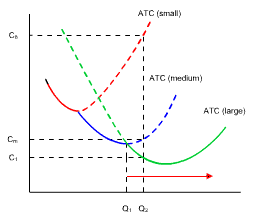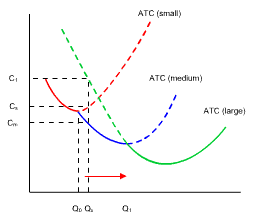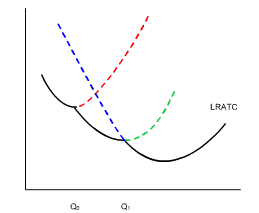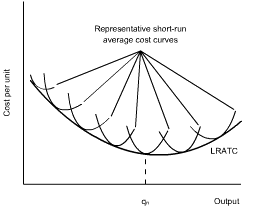#### Subject 8. Understanding Economies and Diseconomies of Scale

Short-Run Cost and Long-Run Cost

The short-run analysis relates costs to output for a specific size of plant. In the long-run, all resources used by the firm are variable.

For each plant size, there is a set of short-run, U-shaped costs curves for MC, AVC, and ATC. This diagram shows the ATC curves of three (of many) possible plant sizes: small, medium, and large.Using this information, firms can plan, when in their blueprint stages, the optimal plant size they should be relative to the output they want to produce. For example, if a firm wanted to produce more than Q1 units of output, it would make sense to build a large firm, since costs per unit would be less than they would be with a small or medium firm.Long-Run Average Cost Curve

To explain this process, imagine the output level Q2. Looking at the relevant costs on the vertical axis, the large firm is far cheaper per unit than both the small and medium-sized firms.Thus, should a firm be planning for output in excess of Q1, a large firm should be built. For levels of output between Q0 and Q1, it would be cheaper per unit if the firm was of a medium size.

• If a firm is planning to produce less than Q0 units, a small firm would be best.
• For output between Q0 - Q1 units, a medium firm is preferable.
• For output in excess of Q1 units, a large firm is preferableThe long-run average total cost curve is indicated in black.It shows the minimum average cost of producing each output level when the firm is free to choose among all possible plant sizes. It can best be thought of as a planning curve, because it reflects the expected per-unit cost of producing alternative rates of output while plants are still in the blueprint stage. No single plant size could produce the alternative output rates at the costs indicated by the planning curve.

In reality, there are an infinite number of firm sizes:The minimum point on the long-run average total cost curve defines the minimum efficient scale for a firm.

Economies and Diseconomies of Scale

Economies of scale are reductions in the firm's per-unit costs that are associated with the use of large plants to produce a large volume of output. They are present over the initial range of outputs when the long-run ATC curve is falling. There are three reasons why economies of scale exist:

• Mass production is more economical.
• Specialization of labor and equipment improves productivity.
• Workers at a larger firm tend to learn more from their experience.
• Bargaining power in input price.

Diseconomies of scale are situations in which the long-run average total costs are greater in larger firms than they are for smaller firms. They are possible: as a firm gets bigger and bigger, bureaucratic inefficiencies may result; principal-agent problems grow; communication breakdowns and bottlenecks can raise input prices. They are present when the long-run ATC curve is rising.

It is also possible to have constant unit costs as the plant size changes. This is known as constant returns to scale.

Economies and diseconomies of scale are long-run concepts. They relate to conditions of production when all factors are variable. In contrast, increased and diminishing returns are short-run concepts, applicable only when the firm has a fixed factor of production.The downward-sloping portion shows economies of scale. The horizontal portion shows constant returns to scale. The upward-sloping portion shows diseconomies of scale.

Minimum efficient scale is the smallest quantity of output at which the long-run average cost reaches its lowest level. If the long-run average cost curve is U-shaped, the minimum point identifies the minimum efficient scale output level.